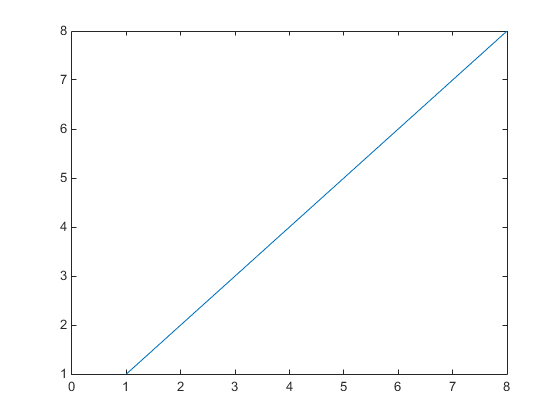## Default Values for Automatically Calculated Properties

### What Are Automatically Calculated Properties

When you create a graph, MATLAB® sets certain property values appropriately for the particular graph. These properties, such as those controlling axis limits and the figure renderer, have an associated mode property.

The mode property determines if MATLAB calculates a value for the property (mode is `auto`) or if the property uses a specified value (mode is `manual`).

### Default Values for Automatically Calculated Properties

Defining a default value for an automatically calculated property requires two steps:

• Define the property default value

• Define the default value of the mode property as `manual`

#### Setting X-Axis Limits

Suppose you want to define default values for the x-axis limits. Because the axes `XLim` property is usually automatically calculated, you must set the associated mode property (`XLimMode`) to `manual`.

```set(groot,'defaultAxesXLim',[0 8]) set(groot,'defaultAxesXLimMode','manual') plot(1:20)```

The axes uses the default x-axis limits of `[0 8]`: